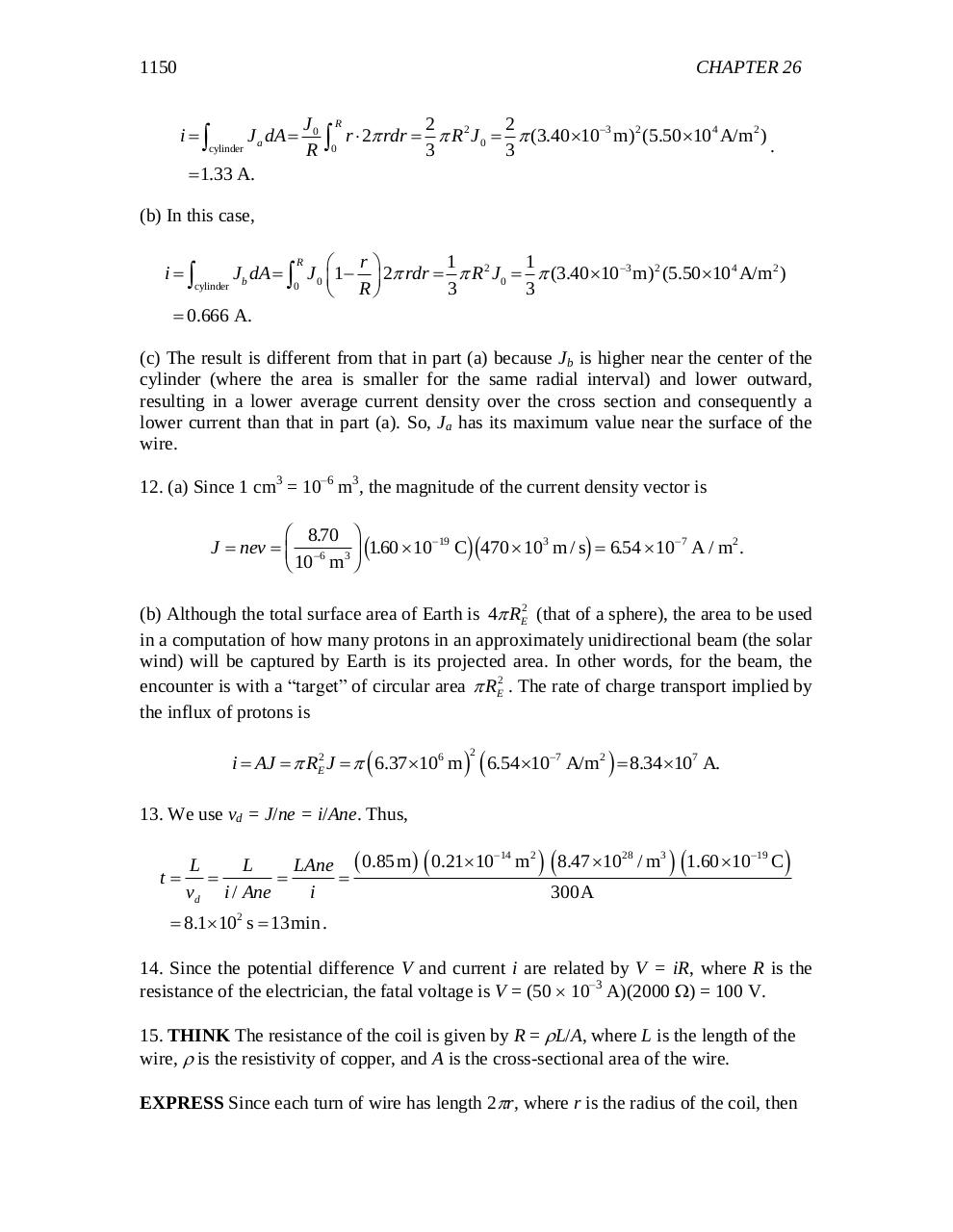# chapter 26.pdfPage 1 2 3 45626

#### Text preview

1150

CHAPTER 26
i

cylinder

J a dA 

J0
R

R

0

2
2
r  2 rdr   R 2 J 0   (3.40 103 m) 2 (5.50 104 A/m2 )
.
3
3

 1.33 A.

(b) In this case,
R
1
1
 r
J b dA   J 0 1   2 rdr   R 2 J 0   (3.40 103 m) 2 (5.50 104 A/m2 )
cylinder
0
3
3
 R
 0.666 A.

i

(c) The result is different from that in part (a) because Jb is higher near the center of the
cylinder (where the area is smaller for the same radial interval) and lower outward,
resulting in a lower average current density over the cross section and consequently a
lower current than that in part (a). So, Ja has its maximum value near the surface of the
wire.
12. (a) Since 1 cm3 = 10–6 m3, the magnitude of the current density vector is

J  nev 

FG 8.70 IJ c160
H 10 m K .  10 Chc470  10 m / sh  6.54  10
19

6

3

3

7

A / m2 .

(b) Although the total surface area of Earth is 4 RE2 (that of a sphere), the area to be used
in a computation of how many protons in an approximately unidirectional beam (the solar
wind) will be captured by Earth is its projected area. In other words, for the beam, the
encounter is with a “target” of circular area  RE2 . The rate of charge transport implied by
the influx of protons is

i  AJ   RE2 J    6.37 106 m   6.54 107 A/m2   8.34 107 A.
2

13. We use vd = J/ne = i/Ane. Thus,
t

14
2
28
3
19
L
L
LAne  0.85m   0.2110 m   8.47 10 / m  1.60 10 C 

vd i / Ane
i
300A

 8.1102 s  13min .

14. Since the potential difference V and current i are related by V = iR, where R is the
resistance of the electrician, the fatal voltage is V = (50  10–3 A)(2000 ) = 100 V.
15. THINK The resistance of the coil is given by R = L/A, where L is the length of the
wire,  is the resistivity of copper, and A is the cross-sectional area of the wire.
EXPRESS Since each turn of wire has length 2r, where r is the radius of the coil, then